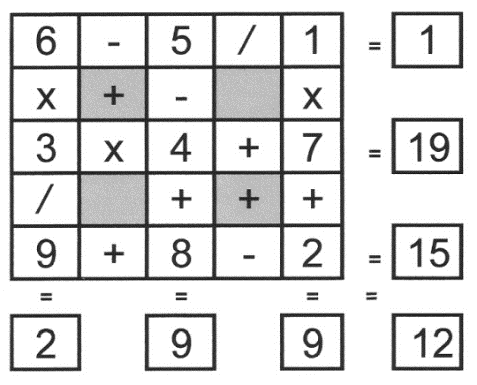Select Page

Each month, a new set of puzzles will be posted.  Come back next month for the solutions and a new set of puzzles, or subscribe to have them sent directly to you.

## MIND-Xpander maths puzzle

Four holes are drilled in a straight line in a rectangular steel plate. The distance between hole 1 and 4 is 35 mm. The distance between hole 2 and hole 3 is twice the distance between hole 1 and hole 2. The distance between hole 3 and hole 4 is the same as the distance between hole 2 and hole 3. What is the distance in milli-metres, between hole 1 and hole 3?

## Can-you-figure-it-out puzzle

How many squares, of any size, are there in the following diagram?## EQUATE+0 puzzle

Each row, column & diagonal is an equation and you use the numbers 1 to 9 to complete the equations. Each number can be used only once. ‘No’ numbers have been provided to get you started. Find the remaining nine numbers that satisfies all the resulting equations. Note – multiplication (x) & division (/) are performed before addition (+) and subtraction (-).## Feedback

There are more than one way of doing these puzzles and may well be more than one answer.  Please let me and others know what alternatives you find by commenting below.  We also welcome general comments on the subject and any feedback you'd like to give.

If you have a question that needs a response from me or you would like to contact me privately, please use the contact form.

## Get more puzzles!

If you've enjoyed doing the puzzles, consider ordering the book; 150+ of the best puzzles in a handy pocket sized format. Click here for full details.

## MIND-Xpander Logic Problem

What value do you get when you sum together the square of the first seven prime numbers?

Answer: 2² + 3² + 5² + 7² + 11² + 13² + 17² = 666

Note: The number 666 in our world today has gotten a bad rap. For many people, seeing this number, or even saying it evokes fear, uncertainty, and mental images of the darkest of forms. 666 comes from Revelation 13 in the Bible where it is referenced as a human number that relates to the ‘mark of the beast’ or devil with 666 blasphemous names.

## Alphametic Puzzles

Alphametic puzzles (sometimes known as Cryptarithms or Verbal Arithmetic), are puzzles where words or phrases are put together in a correct arithmetic expression such that numbers can be substituted for the letters to make the expression true. Find the numeric equivalent for each of the following alphametic expressions. Each letter is unique with its whole-number being 0 to 9.

Solutions## EQUATE+2 Puzzle

Each row, column & diagonal is an equation and you use the numbers 1 to 9 to complete the equations. Each number can be used only once.  One number has been provided to get you started. Find the remaining eight numbers that satisfy all the resulting equations. Note – multiplication (x) & division (/) are performed before addition (+) and subtraction (-).

Solution